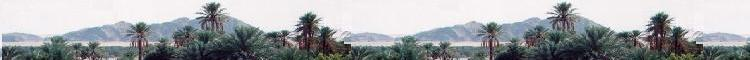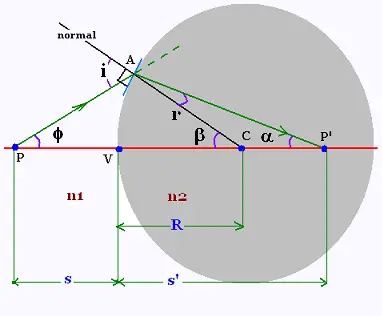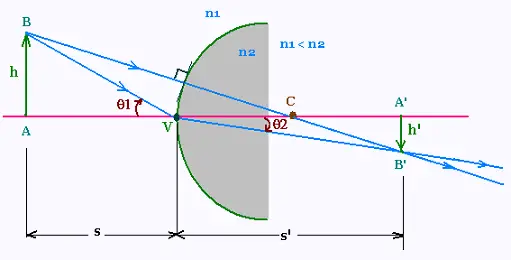##### Optics

Contents

ScientificSentence

# Optics: Images formed by refraction

### 1. Image of a point formed by refractionThe paraxial ray PA refracts and becomes AP'. All the related angles are small; then their sin is equal to their measure radian. The Snell's law is then written as: n1 i = n2 r

We have:
φ + β = i
β = r + α

n1 (φ + β ) = n2 (β - α)
(n2 - n1) β = n1 φ + n2 α

φ = AV/s
α = AV/s'
β = AV/R

(n2 - n1)/R = n1/s + n2/s'

(n2 - n1)/R = n1/s + n2/s'     (1)

### 2. Image of an object formed by refractionWe want to know the lateral magnification m for the object AB of image A'B' provided by the spherical surface.

We have:
tan θ1 = h/s, and tan θ2 = - h'/s'

For small angles, tan θ1 = sin θ1 , and tan θ2 = sin θ2 . Using the law of refraction: n1 sin θ1 = n2 sin θ2, we obtain:
n1 tan θ1 = n2 tan θ2
n1 (h/s) = n2 (-h'/s')

Therefore:
m = h'/h = - n1 s'/n2 s

Lateral magnification - Spherical refracting surface:

m = h'/h = - n1 s'/n2 s     (2)

### 3. Special case: Refraction by flat surface

In the particular case of a plane surace between the two optical materials, the radius of curvature R is infinite. The formula (1) is then written as:

(n2 - n1)/R = n1/s + n2/s' = 0

Therefore:
n1/s = - n2/s' , or
s'/s = - n2/n1

The formula (2) becomes:
h'/h = - n1 s'/n2 s = 1

The image formed by a plane refracting surface has the same lateral size as the object.

Lateral magnification - Plane refracting surface:

m = h'/h = 1

Example: Apparent depth under water

 chimie labs | Physics and Measurements | Probability & Statistics | Combinatorics - Probability | Chimie | Optics | contact |